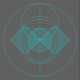This object is in archive!

# Forced rounding create precision troubles

gvitalie shared this problem 4 years ago
New

Good morning, Dear GeoGebra and GeoGebrians.

Forced rounding create precision troubles when need to exact draw values with periodic digits for: segments, squares, areas, etc.

According to Pythagoras Theorem, we should sum areas of two squares something like that.

It should be 0.999..., if 2/9 + 7/9 = 0.222... + 0.777... = 0.999...

See attached picture for details, there we could see that area is equal one instead of 0.999....

Thank You.2

calculate with 32/64 bit in decimal is alway an approximation.

Usual is with rounding and in the most applications this is more exact (see attachment).

If you want cut instead of round for your division you can use

floor(n / 9 (10¹⁵)) / 10¹⁵

.1

Good morning, Dear @rami.

I've understood what's the explanation: it's difference of numerical representation with finite precision and infinite precision.

Example:

- with FINITE precision we'll always have: 1/9 + 8/9 = 0.999... until maximum precision resolution

and when we do rounding, we compensate that finite precision resolution.

- with INFINITE precision we'll always have 1/9 + 8/9 = 1